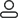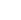LanguageLogin|Sign up
Blog >> Blog Details Page
Engineer Summary: Signal Integrity Design Experience 100 (2)
Posted:02:58 PM August 07, 2018 writer: G

51. The far-end capacitive load increases the rise time of the signal. The 10-90 rise time is approximately (100xC) PS, where C is in PF.

52. If the mutated capacitance is less than 0.004XRT, there may be no problem.

53. The capacitance (Ff) of the corner in the 50 ohm transmission line is twice the line width (MIL).

54. Capacitive mutations increase the delay of 50% points by approximately 0.5XZ0XC.

55. If the abrupt inductance (NH) is less than 10 times the rise time (NS), no problem will occur.

56. For signals with a rise time of less than 1 NS, an axial pin resistance with a loop inductance of approximately 10 NH may produce more reflected noise, which can be replaced by a chip resistor.

57. In a 50 ohm system, a 4 PF capacitor is required to compensate for the 10NH inductance.

At 1 GHz, the resistance of a 1 ounce copper wire is about 15 times that of its DC state.

At 1 GHz, the resistance of the 8 mil wide line produces an attenuation comparable to that produced by the material of this medium, and the attenuation produced by the dielectric material changes more rapidly with frequency.

60. For 3 MIL or wider lines, the low loss state is all above the 10 MHz frequency. In the low loss state, the characteristic impedance and signal speed are independent of loss and frequency. There is no dispersion caused by loss in the common level interconnection.

61 . -3DB Attenuation is equivalent to reducing the initial signal power to 50% and reducing the initial voltage amplitude to 70%.

62 . -20DB attenuation is equivalent to reducing the initial signal power to 1% and reducing the initial voltage amplitude to 10%.

63. When in the skin effect state, the signal path is connected in series with the unit length of the return path (8/W) Xsqrt(f) (where line width W: MIL; frequency F: GHZ).

64. In a 50 ohm transmission line, the unit length attenuation produced by the conductor is approximately 36/(Wz0)DB/IN.

65. The dissipation factor for FR4 is approximately 0.02.

At 66 GHz, the attenuation produced by the dielectric material in FR4 is approximately 0.1 DB/IN and increases linearly with frequency.

67. For 8MIL wide in FR4

68. Constrained by the loss factor, the bandwidth of the FR4 interconnect (which is LEN long) is approximately 30 GHz/LEN.

69. The shortest time that the FR4 interconnect can travel is 10PS/INxLEN.

70. If the interconnect length (IN) is greater than 50 times the rise time (NS), the rising edge degradation caused by loss in the FR4 dielectric board is not negligible.

71. In a pair of 50 ohm microstrip transmission lines, the coupling capacitance between the signal lines is about 5% when the line spacing is equal to the line width.

72. In a pair of 50 ohm microstrip transmission lines, when the line spacing is equal to the line width, the coupling inductance between the signal lines is about 15%.

73. For the rise time of 1NS, the saturation length of the near-end noise in FR4 is 6IN, which is proportional to the rise time.

74. The load capacitance of a line is a constant, independent of the proximity of other lines nearby.

75. For 50 ohm microstrip lines, the near-end crosstalk is approximately 5% when the line spacing is equal to the line width.

76. For 50 ohm microstrip lines, the near-end crosstalk is approximately 2% when the line spacing is 2 times the line width.

77. For a 50 ohm microstrip line, the near-end crosstalk is approximately 1% when the line spacing is 3 times the line width.

78. For a 50 ohm stripline, the near-end crosstalk is approximately 6% when the line spacing is equal to the line width.

79. For a 50 ohm stripline, the near-end crosstalk is approximately 2% when the line spacing is 2 times the line width.

80. For a 50 ohm stripline, when the line spacing is 3 times the line width, the near-end crosstalk is about 0.5%.

81. In a pair of 50 ohm microstrip transmission lines, the far-end noise is 4% Xtd/rt when the spacing is equal to the line width. If the line delay is 1 ns and the rise time is 0.5 ns, the far-end noise is 8%.

82. In a pair of 50 ohm microstrip transmission lines, the far-end noise is 2% Xtd/rt when the spacing is 2 times the line width. If the line delay is 1 ns and the rise time is 0.5 ns, the far-end noise is 4%.

83. In a pair of 50 ohm microstrip transmission lines, when the spacing is 3 times the line width, the far-end noise is 1.5% Xtd/rt. If the line delay is 1 ns and the rise time is 0.5 ns, the far-end noise is 4%.

84. There is no far-end noise on the stripline or fully embedded microstrip line.

85. In a 50 ohm bus, whether it is a stripline or a microstrip line, to minimize the far-end noise below 5%, you must maintain a line spacing greater than 2 times the line width.

86. In a 50 ohm bus, when the line-to-line distance is equal to the line width, 75% of the nuisance on the victim line comes from the two followers on either side of the victim line.

87. In a 50 ohm bus, when the line distance is equal to the line width, 95% of the nuisance on the victim line comes from the two lines on each side of the victim line that are closest to each other.

88. In a 50 ohm bus, when the line distance is twice the line width, 100% of the nuisance on the victim line comes from the two lines adjacent to the two sides of the victim line. This is to ignore the coupling to all other lines in the bus.

89. For surface wiring, increase the distance between adjacent signal lines to make it enough to add a protective wiring, crosstalk is often reduced to an acceptable level, and it is not necessary to increase the protective wiring. Adding a shorted protective wiring to the terminal reduces crosstalk to 50%.

90. For striplines, use a guard wire to reduce crosstalk to 10% when the guard cord is not used.

91. In order to keep the switching noise at an acceptable level, the mutual inductance must be less than 2.5nhx rise time (ns).

92. For connectors or packages that are subject to switching noise limitations, the maximum available clock frequency is 250 MHz/(NxLm). Among them, Lm is the mutual inductance (nh) between the signal/return path pairs, and N is the number of simultaneous opening.

93. In LVDS signals, the common mode signal component is more than 2 times larger than the differential signal component.

94. If there is no coupling between them, the differential impedance of the differential pair is twice the impedance of any one of the single-ended lines.

95. A pair of 50 ohm microstrip lines, as long as the voltage of one of the lines remains high or low, the single-ended characteristic impedance of the other line is completely independent of the distance from the adjacent line.

96. In a tightly coupled differential microstrip line, the differential characteristic impedance is only reduced by about 10% when the line is far apart and there is no coupling compared to the coupling when the line width is equal to the line spacing.

97. For wide-edge coupled differential pairs, the distance between the lines should be at least greater than the line width. The purpose of this is to obtain a tap impedance of up to 100 ohms.

98. The FCC's Class B requirement is that at 100 MHz, the far field strength at 3M is less than 150 UV/M.

99. The adjacent single-ended attack secondary line produces 30% less differential signal crosstalk on the strongly coupled differential pair than on the weakly coupled differential pair.

100. The adjacent single-ended attack secondary line produces 30% more common-mode signal crosstalk on the strongly coupled differential pair than on the weakly coupled differential pair.

567 1 0 1
• PCB
Prototype
• PCB
Assembly
• SMD
Stencil
 Dimensions: (mm) × Quantity: (pcs) 5 5 10 15 20 25 30 40 50 75 100 120 150 200 250 300 350 400 450 500 600 700 800 900 1000 1500 2000 2500 3000 3500 4000 4500 5000 5500 6000 6500 7000 7500 8000 9000 10000 Other Quantities:(quantity*length*width is greater than 10㎡) OK Layers: 1 2 4 6 8 10 12 Thickness: 0.6 mm 0.8 mm 1.0 mm 1.2 mm 1.6 mm 2.0 mm 2.5 mmQuote now